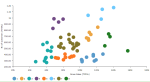# DCPY.KMEANSCLUST(n_clusters, random_state, init, n_init, max_iter, columns)

The K-means algorithm is one of the most popular general clustering algorithm. It computes K centroids that it uses to define clusters. A point is considered to be in a particular cluster if it is closer to that cluster's centroid than to any other centroid. K-means finds the best centroids by alternating between assigning data points to clusters based on the current centroids and recalculating centroids based on the current assignment of data points to clusters. For measuring how far a data point is from other centroids, Euclidean distance is used.

###### Parameters
• n_clusters – Number of clusters that the algorithm should find, integer (default 8).

• random_state – Seed used to generate random numbers, integer (default 0).

• init – Method for initializing the centroids (default 'k-means++'):

• k-means++ – First initial centroid is selected at random, other centroids are selected to be as far apart from each other as possible. This method speeds up the convergence and helps to avoid converging at local optimum.
• random – Centroids are initially chosen randomly from input data points.
• n_init – Number of times that the K-means algorithm is run with different centroid seeds. The final result is the one with the lowest within-cluster sum-of-squares (inertia), integer (default 10).

• max_iter – Maximum number of iterations (recalculations of centroids) in a single run, integer (default 300).

Example: DCPY.KMEANSCLUST(8, 0,'k-means++', 10, 300, sum([Gross Sales]), sum([No of customers])) used as a calculation for the Color field of the Scatterplot visualization.###### Input data
• Numeric variables are automatically scaled to zero mean and unit variance.
• Character variables are transformed to numeric values using one-hot encoding.
• Dates are treated as character variables, so they are also one-hot encoded.
• Size of input data is not limited, but many categories in character or date variables increase rapidly the dimensionality.
• Rows that contain missing values in any of their columns are dropped.
###### Result
• Column of integer values starting with 0, where each number corresponds to a cluster assigned to each record (row) by the K-means algorithm.
• Rows that were dropped from input data due to containing missing values have missing values instead of assigned cluster.
###### Key usage points
• Fast and computationally efficient, scalable.
• Practically works well, even if some of its assumptions are broken.
• General-purpose clustering algorithm.
• Use it when the approximate number of clusters is known.
• Sensitive to outliers. Use it if data has low number of outliers.
• Use it when the clusters are spherical, with approximately same number of observations, density and variance.
• Euclidean distances tend to be more inflated with higher number of variables (curse of dimensionality).
• By calculating Euclidean distance, the algorithm makes assumption of only numeric input variables. One-hot encoding of categorical variables is a workaround suitable for relatively low number of categories to encode.
• K-means makes the assumption that we deal with spherical clusters and that each cluster has roughly equal numbers of observations, density, and variance; otherwise, the results might be misleading.
• Always finds clusters in the data, even if no natural clusters are present.
• All data points are assigned to a cluster, even if some of them might be just random noise.
###### Example

For the whole list of algorithms, see Data science built-in algorithms.